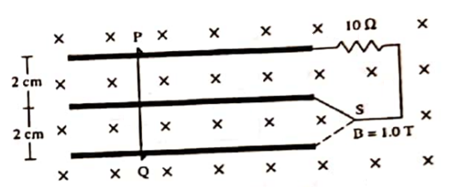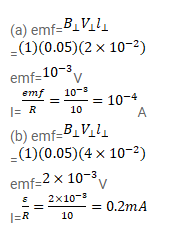# Consider the situation shown in figure.

Question:

Consider the situation shown in figure. The wire PQ has a negligible resistance and is made to slide on the three rails with a constant speed of $5 \mathrm{~cm} / \mathrm{s}$. Find the current in the $10 \Omega$ resistor when the switch $\mathrm{S}$ is thrown to

(a) the middle rail

(b) the bottom rail.Solution: